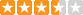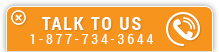Statics: Vector Mechanics

Course Number: S-4012
Credit: 4 PDH
Subject Matter Expert: Mark A. Strain, P.E.
Price: \$119.80 Purchase using Reward Tokens. Details54 reviews
Overview

In Statics: Vector Mechanics, you'll learn ...

• How to use a free body diagram to calculate static forces
• How to determine forces in a pulley system
• How to determine the internal forces in truss members
• How to calculate static and dynamic frictional forces

OverviewPreview a portion of this course before purchasing it.

Credit: 4 PDH

Length: 35 pages

Mechanics is the branch of science concerned with the behavior of physical bodies when subjected to forces or displacements, and the effects of the bodies on their environment. Mechanics is a physical science incorporating mathematical concepts directly applicable to many fields of engineering such as mechanical, civil, structural and electrical engineering.

Vector analysis is a mathematical tool used in mechanics to explain and predict physical phenomena. The word “vector” comes from the Latin word vectus (or vehere – meaning to carry). A vector is a depiction or symbol showing movement or a force carried from point A to point B.

Statics (or vector mechanics) is the branch of mechanics that is concerned with the analysis of loads (or forces and moments) on physical systems in static equilibrium. Systems that are in static equilibrium are either at rest or the system's center of mass moves at a constant velocity. Unlike dynamics, where the components of the system are in motion, components of a system in static equilibrium do not move or vary in position relative to one another over time. Problems involving statics use trigonometry to find a solution.

Newton's First Law states that an object at rest tends to stay at rest or an object in motion tends to stay in motion at a constant velocity, unless acted upon by an external force. In the area of statics Newton's First Law dictates that the sum of all forces, or net force, and net moment on every part of the system are both zero.

The term "static" means still or unchanging. In relation to vector mechanics the terms "still" or "unchanging" pertain to the system under evaluation. The system may be at rest or may be moving at a constant velocity, but all of the components of the system are still or in equilibrium with each other. However, there are forces within the system usually acted upon by gravity, but all of the forces are balanced.

This course is intended to be a refresher course for statics (vector mechanics). This course is intended for someone who has a general working knowledge of vectors. This course has a lot of sample problems and teaches by showing examples and sample problems.

Specific Knowledge or Skill Obtained

This course teaches the following specific knowledge and skills:

• How to decompose a force into its vector components
• How to determine the equilibrium of a particle
• How to determine the equilibrium of a rigid body
• How to determine the mechanical advantage of a pulley system
• How bodies are subject to moments (or torque)
• How to reduce a force couple to a moment
• How to reduce a system to a force and a moment
• How to determine if a truss is stable
• How to determine the axial forces within a truss by the method of joints
• How to determine the axial forces within a truss by the method of sections
• How to calculate the force of friction between a body and a surface
• How the determine the equilibrium of a body when the force of friction acts on the body

Certificate of Completion

You will be able to immediately print a certificate of completion after passing a multiple-choice quiz consisting of 30 questions. PDH credits are not awarded until the course is completed and quiz is passed.

Board Acceptance
 This course is applicable to professional engineers in: Alabama (P.E.) Alaska (P.E.) Arkansas (P.E.) Delaware (P.E.) Florida (P.E. Area of Practice) Georgia (P.E.) Idaho (P.E.) Illinois (P.E.) Illinois (S.E.) Indiana (P.E.) Iowa (P.E.) Kansas (P.E.) Kentucky (P.E.) Louisiana (P.E.) Maine (P.E.) Maryland (P.E.) Michigan (P.E.) Minnesota (P.E.) Mississippi (P.E.) Missouri (P.E.) Montana (P.E.) Nebraska (P.E.) Nevada (P.E.) New Hampshire (P.E.) New Jersey (P.E.) New Mexico (P.E.) New York (P.E.) North Carolina (P.E.) North Dakota (P.E.) Ohio (P.E. Self-Paced) Oklahoma (P.E.) Oregon (P.E.) Pennsylvania (P.E.) South Carolina (P.E.) South Dakota (P.E.) Tennessee (P.E.) Texas (P.E.) Utah (P.E.) Vermont (P.E.) Virginia (P.E.) West Virginia (P.E.) Wisconsin (P.E.) Wyoming (P.E.)
Reviews (54)
More DetailsPreview a portion of this course before purchasing it.

Credit: 4 PDH

Length: 35 pages